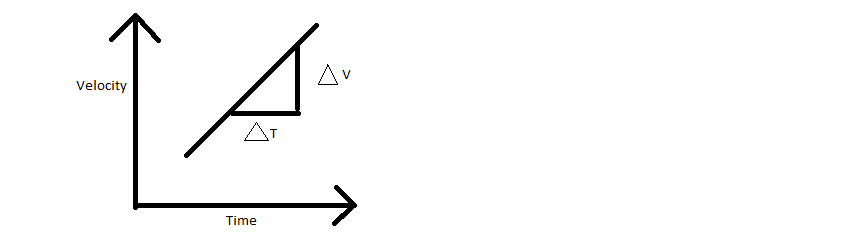# What does the slope of a velocity- time represent?

The slope in the velocity-time graph represents the acceleration.

The slope is defined as the ratio of change in y-axis to change in the x-axis.

The slope is represented by the letter m and following is the general formula used for determining the slope:

 $$m=\frac{y_{2}-y_{1}}{x_{2}-x_{1}}$$

Where,

• m is the slope
• y2 – y1 is the difference in the units on the y-axis
• x2 – x1 is the difference in the units on the x-axis(6)(1)